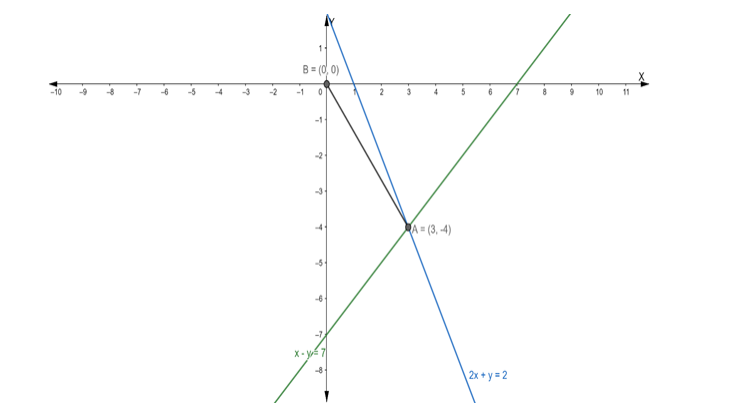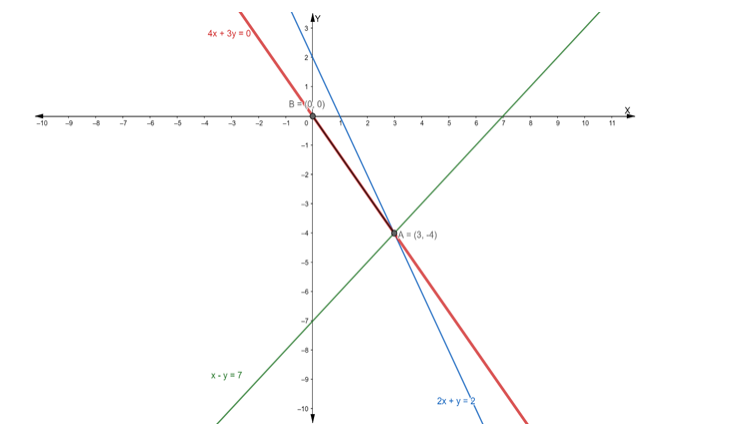# Find the equation of the line drawn through the point of intersection of the`
Question:

Find the equation of the line drawn through the point of intersection of the lines x – y = 7 and 2x + y = 2 and passing through the origin.

Solution:

Suppose the given two lines intersect at a point $P\left(x_{1}, y_{1}\right)$. Then, $\left(x_{1}, y_{1}\right)$ satisfies each of the given equations.

$x-y=7 \ldots$ (i)

$2 x+y=2 \ldots$ (ii)

Now, we find the point of intersection of eq. (i) and (ii)

Multiply the eq. (i) by 2, we get

$2 x-2 y=14 \ldots$ (iii)

On subtracting eq. (iii) from (ii), we get

$2 x-2 y-2 x-y=14-2$

$\Rightarrow-3 y=12$

$\Rightarrow y=-4$

Putting the value of y in eq. (i), we get

$x-(-4)=7$

$\Rightarrow x+4=7$

$\Rightarrow x=7-4$

$\Rightarrow x=3$

Hence, the point of intersection $\mathrm{P}\left(\mathrm{x}_{1}, \mathrm{y}_{1}\right)$ is $(3,-4)$Let $A B$ is the line drawn from the point of intersection $(3,-4)$ and passing through the origin.

Firstly, we find the slope of the line joining the points $(3,-4)$ and $(0,0)$

Slope of line joining two points $=\frac{y_{2}-y_{1}}{x_{2}-x_{1}}$

$\therefore \mathrm{m}_{\mathrm{AB}}=\frac{0-(-4)}{0-3}=\frac{4}{-3}$

Now, we have to find the equation of the line passing through the origin

Equation of line: $y-y_{1}=m\left(x-x_{1}\right)$

$\Rightarrow \mathrm{y}-0=-\frac{4}{3}(\mathrm{x}-0)$

$\Rightarrow 3 \mathrm{y}=-4 \mathrm{x}$

$\Rightarrow 4 \mathrm{x}+3 \mathrm{y}=0$

Hence, the equation of the line passing through the origin is $4 x+3 y=0$